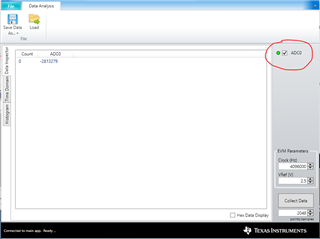If you have a related question, please click the "Ask a related question" button in the top right corner. The newly created question will be automatically linked to this question.

Hi Team,

Page 30 of the Delta-Sigma ADC EvaluaTIon Software User Manual shows 4 ADC channels (AD0-AD3). Our customer would like to know how to enable these four ADC channels as his GUI shows only 1 channel. Please see the screenshot below for reference.Regards,

Danilo

• Hi Danilo,

Some ADCs have multiple ADCs for simultaneous sampling.  The ADS124S08 has only a single ADC and a mux that is in front of it.  So this device does not allow multiple conversion data to be presented in a single plot within the GUI as it only has one ADC.

Best regards,

Bob B.

• Hi Bob,

Thank you for this information. Our customer has a follow up inquiry below.

I have an external voltage (2.0V) connected to AIN0. How do I configure the GUI to get the ADC monitor value? Will GUI report 2.0V, or what is the formula should I use to convert the register hex value to voltage?

Regards,

Danilo

• Hi Danilo,

On the same Analysis Client page you show in the original post there is a box labeled VRef.  Change this value (2.5V default) to the reference voltage being used and the GUI will convert the code to volts.

Otherwise the code to volt conversion is given in the ADS124S08 datasheet. The value of 1 code is equal to 2 * Vref / Gain * (2^24 - 1).

Best regards,

Bob B

• I have an external voltage connected to AIN10, how to configure the GUI (INPMUX + others) so I can use "Data Analysis" to see if ADC reports the same voltage?

• Hi Tony,

See section 5.1.3 in the ADS124S08EVM user's guide for connection assignment information.  Note that this connection uses a voltage divider so the measurement is indirect.  The actual voltage input to the ADC uses formula 16 as given in the user's guide.  This type of connection demonstrates how to connect an external voltage greater than the analog supply.  If you want a direct voltage measurement you need to use a different ADC input as AIN10 is dedicated to the voltage divider.

Best regards,

Bob B

• Below is my J9 sensor connection, and the external voltage connected to AIN10 is 2.5V.

0-24V (REF = 5V): AVDD = 5V, AINCOM = AGND, AINP = AIN10, AINN = AINCOM.

The measurement reported by GUI is 856564. Questions:

1. Is this value (856564) correct?

2. How to convert this value to voltage?

• Another question: I have a differential voltages (example V1=1.5V, V2=1.0V). Which AIN channels should I use?

• Hi Tony,

When using the voltage divider circuit of R76 and R77 and then attempting to convert the ADC code to the input voltage requires a couple of steps.  The first is to calculate the measured voltage across R77.  To do this you need to determine the value of one code.  The value of 1 LSB (or 1 ADC code) uses the formula of (2 * VREF) / (Gain * (2^24 - 1).  If a gain of 1 is used along with a 5V VREF the equation reduces to 5V / (2^23) or approximately 596nV per code.

To get the value of the voltage across the inputs (or across R77 in this case) you multiply the LSB value times the number of codes from the ADC.  If the result is 856564, the voltage is 856564 * 596nV which is approximately 510.55mV.

As there is a voltage divider at the input, the input voltage is equal to the ADC voltage result (510.55mV) times (R76 + R77) / R77.  Or 510.55nV * 117.1 / 24 which is approximately equal to 2.4911V.

1. Is this value (856564) correct?

It would seem that the voltage is very close.  We are assuming that the input voltage is exactly 2.5V, the reference is exactly 5.0V and the resistors are ideal values.  So without measuring all of the values and recalculating the result I would say this is fairly accurate.

2. How to convert this value to voltage?

The calculation is as I stated above.

Another question: I have a differential voltages (example V1=1.5V, V2=1.0V). Which AIN channels should I use?

As AVDD is 5V, you can connect the inputs directly as they are both less than 5V.  Some of the EVM inputs make a direct measurement difficult as the inputs may be assigned to a special purpose.  Mostly likely you would want to connect the inputs to J8  at A8 or A9 or any of the inputs on J7.

Best regards,

Bob B